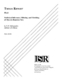## Statistical Inference, Filtering, and Modeling of Discrete Random Sets

 dc.contributor.advisor Baras, J.S. en_US dc.contributor.author Sidiropoulos, N.D. en_US dc.contributor.department ISR en_US dc.date.accessioned 2007-05-23T09:52:27Z dc.date.available 2007-05-23T09:52:27Z dc.date.issued 1992 en_US dc.description.abstract The objective of this dissertation is the systematic study of several aspects of modeling, statistical inference, and filtering of "random" binary digital images, or, uniformly bounded discrete random sets. This study consists of two interleaved parts. In the first part, we consider some important aspects of a theory of uniformly bounded discrete random sets. The fundamental result is a strengthened version of a backbone theorem of continuous- domain random set theory, namely the uniqueness theorem of Choquet-Kendall-Matheron, for the case of uniformly bounded discrete random sets.

The vehicle through which much of the discussion is carried out is a "special" discrete random set model, the discrete radial Boolean random set, which is closely related to the theory of Morphological shape-size distributions. The continuous-domain Boolean random set has been successfully used in a variety of applications. A good portion of the first part of this dissertation is devoted to the statistical inference of the discrete radial Boolean random set. We consider three problems: parameter estimation, binary hypothesis testing, and classification of "random" known shapes in Boolean clutter. The tools come mainly from the area of Morphological shape analysis. The focus is on inference procedures which are both computationally efficient, and statistically sound.

In the second part, we consider the problem of estimating realizations of uniformly bounded discrete random sets, distorted by a degradation process which can be described by a union/intersection noise model. Two different optimal filtering approaches are considered. The first involves a class of filters which arises quite naturally from the set-theoretic analysis of optimal filters. We call this the class of mask filters. The second approach deals with optimal Morphological filters. First, we provide some fresh statistical insight into certain "folk theorems" of Morphological filtering. We do so by exploiting the uniformly bounded discrete random set formulation of the filtering problem. Then we show that, by using an appropriate (under a given degradation model) expansion of the optimal filter, we can obtain "universal" characterizations of optimality, in terms of the fundamental functionals of random set theory, namely the generating functionals of the signal and the noise. en_US dc.format.extent 13037982 bytes dc.format.mimetype application/pdf dc.identifier.uri http://hdl.handle.net/1903/5318 dc.language.iso en_US en_US dc.relation.ispartofseries ISR; PhD 1992-10 en_US dc.subject detection en_US dc.subject estimation en_US dc.subject filtering en_US dc.subject image processing en_US dc.subject information theory en_US dc.subject Systems Integration en_US dc.title Statistical Inference, Filtering, and Modeling of Discrete Random Sets en_US dc.type Dissertation en_US

##### Original bundle
Now showing 1 - 1 of 1